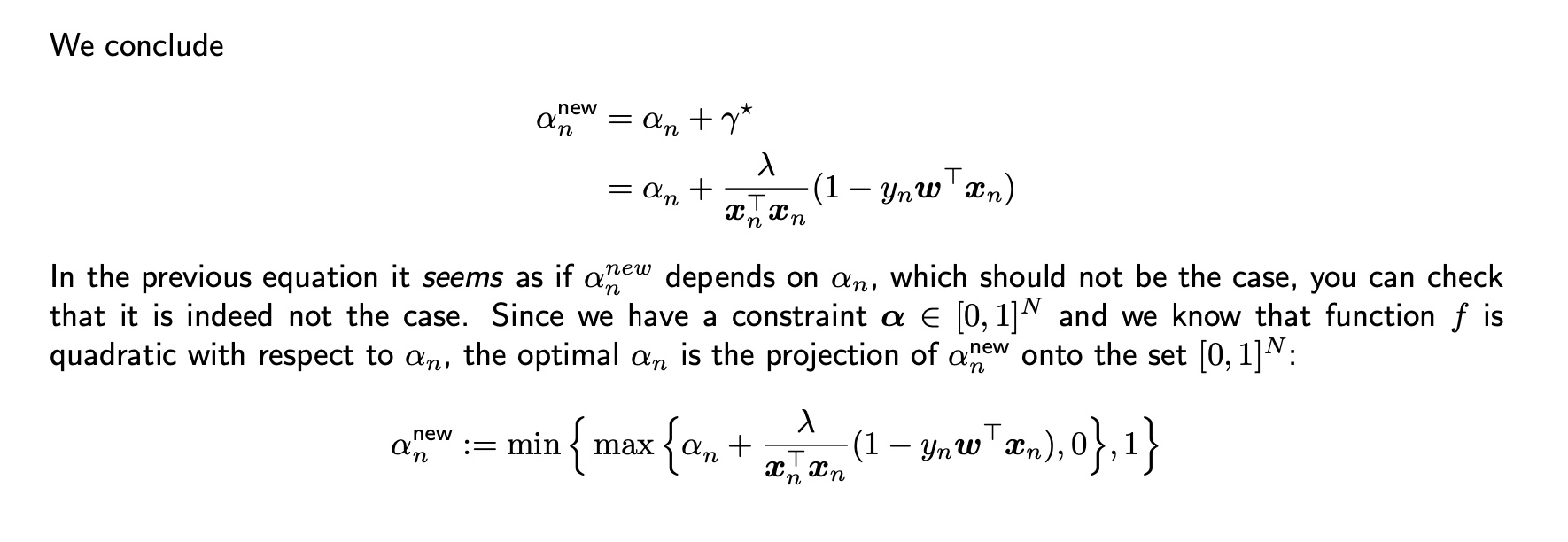### SVM dual optimization

Hello, I had a question regarding the dual formulation ascent step. I do not understand why $$\alpha^{new}$$ should not depend on $$\alpha_{n}$$, and how the fact that f is quadratic and $$\alpha$$ is constrained implies that the optimal $$\alpha$$ is a projection onto the set $$[0, 1]$$.Any further clarification/ intuition would be much appreciated :)

Never mind, I just saw the other post where you have to sub in the expression $$w(\alpha)$$ and the $$\alpha_{n}$$ term drops out. However, I am still unsure about the projection and how the min max statement is obtained, and further how this is done in $$O(D)$$.

Wondering the same things ! Also, could we have written max{min{..., 1}, 0} ? (with concacvity and convexity of w and alpha)

Hi, could anyone elaborate on this please, I still have not figured it out :(

You want alpha to be in interval [0, 1]. If it isn't you just take the closest point in interval [0, 1] instead of it (i.e. you are projecting value of alpha on a convex set, in this case that set is [0, 1] interval). If alpha < 0 then closest point in [0, 1] to alpha is 0, that's why you take the max(alpha, 0). Similarly when alpha > 1 closest point to it is 1, so you take min(alpha, 1). If alpha is in [0, 1] already than min max will just return alpha. Here I considered alpha to be a one dimensional vector but it is the same principal when alpha is N dimensional and constraint set is [0, 1]^N, you just apply the same technique to every component individually (notice n subscript to alpha in their notation).

Page 1 of 1